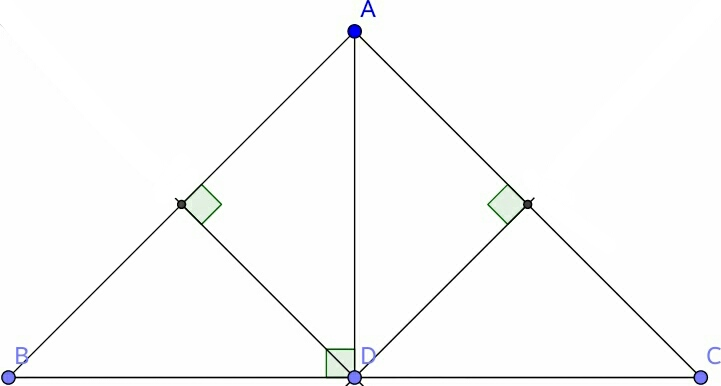# Which center of triangle?

Geometry Level 3In the triangle above, what is point $\text{D}$ with respect to the right isosceles $\triangle{\text{ABC}}$ with $\text{BC}$ as the hypotenuse?

×